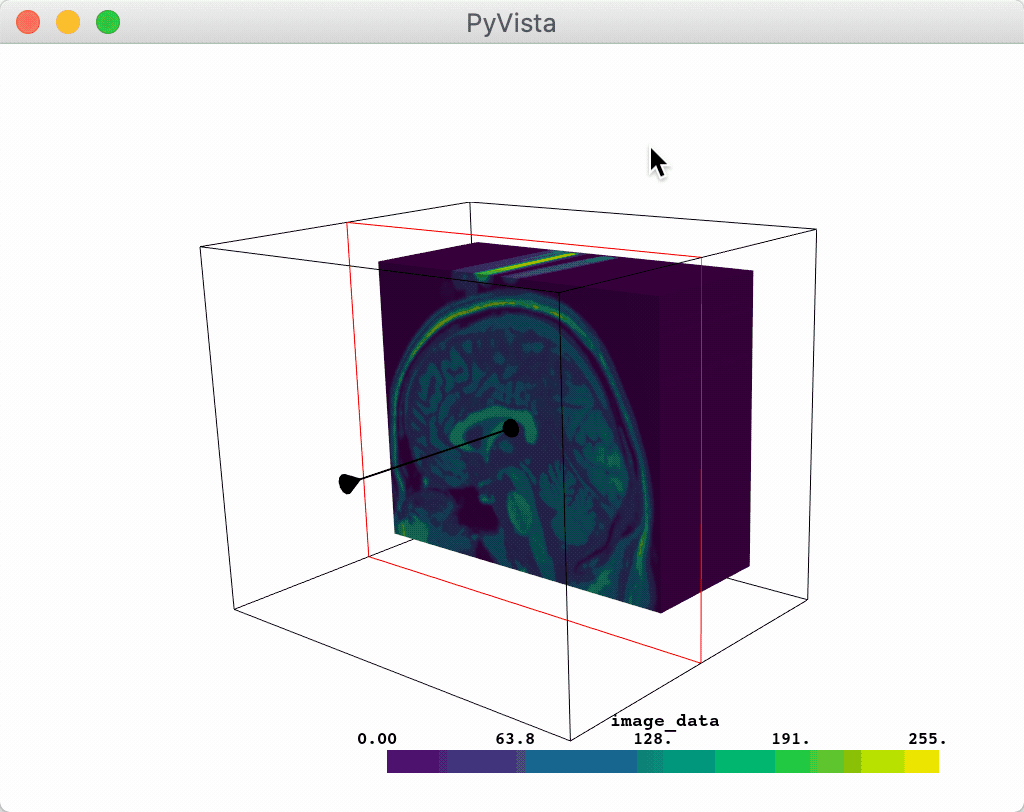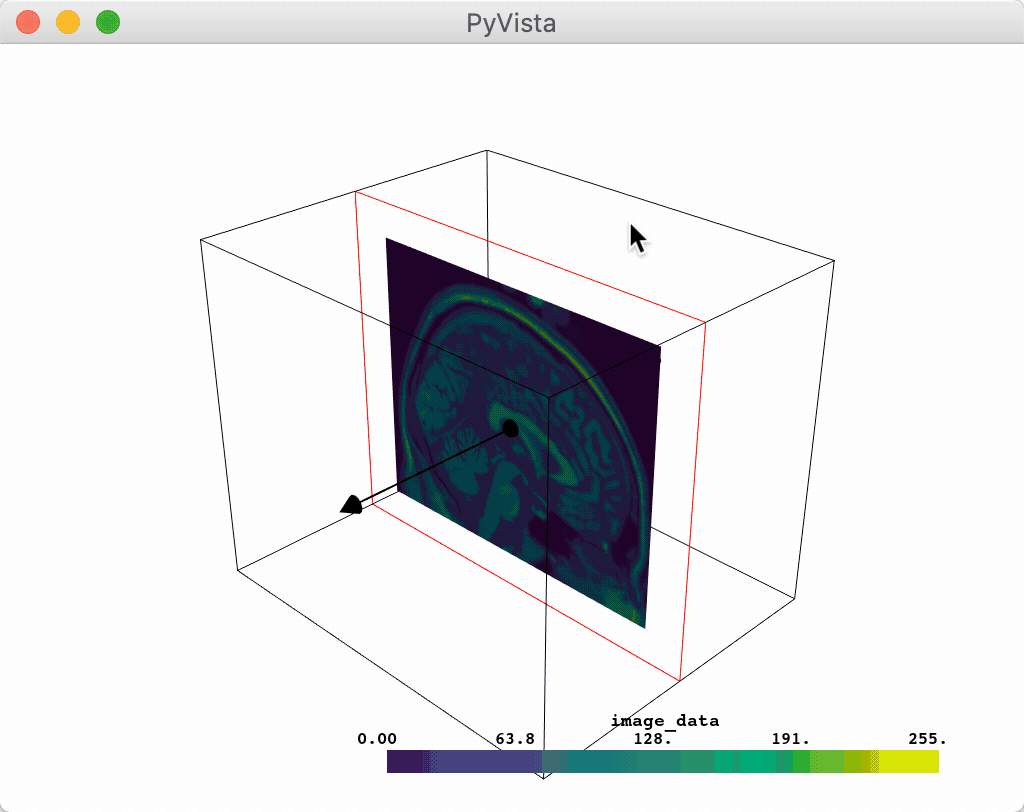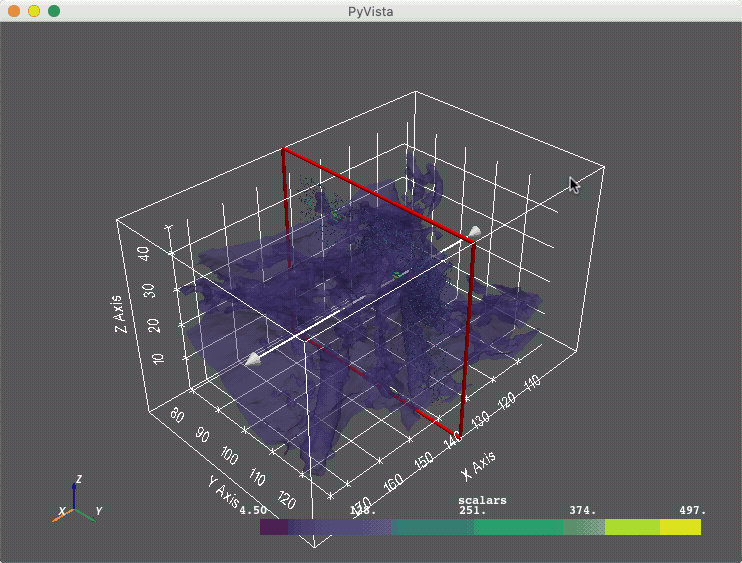# Plane Widget#

The plane widget can be enabled and disabled by the `pyvista.Plotter.add_plane_widget()` and `pyvista.Plotter.clear_plane_widgets()` methods respectively. As with all widgets, you must provide a custom callback method to utilize that plane. Considering that planes are most commonly used for clipping and slicing meshes, we have included two helper methods for doing those tasks!

Let’s use a plane to clip a mesh:

```import pyvista as pv
from pyvista import examples

p = pv.Plotter()
p.show()
```After interacting with the scene, the clipped mesh is available as:

```p.plane_clipped_meshes
```
```[UnstructuredGrid (0x7f5918438c40)
N Cells:    3538080
N Points:   3613484
X Bounds:   9.000e+01, 1.800e+02
Y Bounds:   0.000e+00, 2.160e+02
Z Bounds:   0.000e+00, 1.800e+02
N Arrays:   2
]
```

And here is a screen capture of a user interacting with thisOr you could slice a mesh using the plane widget:

```p = pv.Plotter()
p.show()
```After interacting with the scene, the slice is available as:

```p.plane_sliced_meshes
```
```[PolyData (0x7f58c02f47c0)
N Cells:    38880
N Points:   39277
N Strips:   0
X Bounds:   9.000e+01, 9.000e+01
Y Bounds:   0.000e+00, 2.160e+02
Z Bounds:   0.000e+00, 1.800e+02
N Arrays:   1
]
```

And here is a screen capture of a user interacting with thisOr you could leverage the plane widget for some custom task like glyphing a vector field along that plane. Note that we have to pass a `name` when calling `add_mesh` to ensure that there is only one set of glyphs plotted at a time.

```import pyvista as pv
from pyvista import examples

p = pv.Plotter()

def my_plane_func(normal, origin):
slc = mesh.slice(normal=normal, origin=origin)
arrows = slc.glyph(orient='vectors', scale="scalars", factor=0.01)

p.show_grid()
p.show()
```And here is a screen capture of a user interacting with thisFurther, a user can disable the arrow vector by setting the `normal_rotation` argument to `False`. For example, here we programmatically set the normal vector on which we want to translate the plane and we disable the arrow to prevent its rotation.

```p = pv.Plotter()
p.show()
```The vector is also forcibly disabled anytime the `assign_to_axis` argument is set.

```p = pv.Plotter()
p.show()
```Additionally, users can modify the interaction event that triggers the callback functions handled by the different plane widget helpers through the `interaction_event` keyword argument when available. For example, we can have continuous slicing by using the `InteractionEvent` observer.

```import vtk

p = pv.Plotter()And here is a screen capture of a user interacting with this continuously via the `InteractionEvent` observer: